Next: Some GRT corollaries Up: Criticism of the GRT basis Previous: The equivalence principle   Contents

## Time in GRT

The notion of time in GRT is confused beyond the limit as well. What does it mean by the clock synchronization, if it is possible only along the unclosed lines? The change of the moment of time reference point in moving around a closed path is an obvious contradiction of GRT, since at a great synchronization rate many similar passes-around can be made, and arbitrary aging or rejuvenation can be obtained. For example, considering the vacuum (emptiness) to be rotating (if we ourselves shall move around a circle), we can get various results depending on a mental idea.

If we momentarily believe the GRT dependence of time from the gravitational potential and believe the equivalence of gravitation and non-inertiality (an acceleration), then it could be easily understand that time depends on the relative acceleration in this case (it is an extended interpretation). Really, different accelerations correspond to different gravitational potentials in this case, and conversely. But relative accelerations possess the vector character (and it cannot be "hidden"), that is the extended interpretation is the only possible one. Using the modified paradox of twins , the independence of time on acceleration for extended interpretation can easily be proven. Let two astronauts - the twins - are at a great distance from each other. On a signal of the beacon, situated at the middle, these astronauts begin to fly toward a beacon at the same acceleration (Fig. 2.6).Since in GRT the time depends on the acceleration and the acceleration has relative character, each of the astronauts will believe, that his twin brother is younger than he is. At meeting near the beacon they can exchange photos. However, owing to the problem symmetry, the result is obvious: the time in an accelerated system flows at the same rate, as in non-accelerated one. Besides, each astronaut (third observer can be placed at the beacon) can send the signals to the other one about his each birthday. The same number of light spheres will be perceived by each astronaut till they meet at the beacon (there is nowhere to hide the spheres). Having received a "telegram" about 50th birthday of the brother a minute before the meeting, whether the other astronaut will congratulate the brother on his 5th birthday (maybe, he needs the oculist)? If we suppose the gravitational field to be equivalent to the acceleration (according to GRT), then we obtain, that the time intervals do not depend on the gravitational field presence. For example, the extend interpretation which includes the relationship between time and acceleration can be easily disproved in the following manner. Let us consider several mans in different parts of the Earth. If we will use the GRT equivalence of the gravitational field and an acceleration, then, to imitate the terrestrial attraction, they must be accelerated from the Earth's center, that is in different directions (all acceleration vectors will differ their directions). Therefore, all relative accelerations will be different. Owing to the problem symmetry, the result is obvious: the age of these mans will be independent on their location.

Now we make some remarks concerning the method of synchronization of times by means of a remote periodic source disposed perpendicular to the motion of a body . We begin with inertial systems. The possibility of time synchronization on restricted segments of the trajectory makes it possible to synchronize the time throughout the line of motion (Fig. 2.7).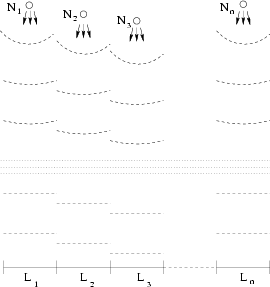Indeed, if for each segment there exists an arbitrarily remote periodic source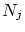sending the following information: its number, the quantity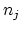of passed seconds (the time reference point is not coordinated with other sources), then the observers at junctions of segments can compare the time reference point for a source on the left and for a source on the right. Transmitting this information sequentially from the first observer to the last one, it is possible to establish a single time reference point (the time itself, as it was shown in Chapter 1, has absolute sense ).

Apparently, the observed rate of transmission of synchronization signals has no effect on the determination of duration of times: the pulses (for example, light spheres or particles), which mark the number of passed seconds, will equidistantly fill the whole space, and the number of spheres emitted by a source will be equal to the number of spheres, which reach the receiving observer. (We are not the gods, you see, to be able to introduce the "beginning of times": the time takes already its normal course and elapses uniformly.) Even if we consider the apparent signal propagation rate to be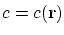, then, irrespective of the path of light, the number of spheres reached the receiving observer (having a zero velocity component in the source direction) will be the same as the number of spheres emitted by a source (simply, the spheres can be spatially thickened or rarefied somewhere). Time as the duration will be perceived uniformly. Thus, the full synchronization is possible in the presence of spatial inhomogeneities (of the gravitational field) as well.

We would remind two well-known experiments which were urgently ascribed by relativists to GRT advantages. The Hafele-Keating's experiment consisted in the following: two pairs of cesium atomic watch flew at an airplane in the east and west directions, and their readings were compared with the resting watch (in so doing the SRT "velocity effect" was taken into consideration, but its lack was proved in Chapter 1 of the present book). The Pound-Rebka's experiment consisted in the following: using the Mossbauer effect, a frequency shift was detected for a photon which passed some distances in the vertical directions (both up and down). In physics it is not accepted to take into account the same effect twice. It is clear, that the acceleration and gravitation express some force, that influences various processes. But this will be the general result of the effect of namely the forces. For example, not any overload can be withstood by a man, the pendulum clock will not operate under zero gravity, but this does not mean, that the time stopped. Therefore, the rough Hafele-Keating's experiment states the trivial fact, that the gravitation and acceleration somehow influence the processes in a cesium atomic watch, and the high relative accuracy of this watch for a fixed site is fully groundless. Besides, interpretation of this experiment contradicts the "explanation" of the Pound-Rebka's experiment with supposition about independence of frequency of emission in "the units of intrinsic atom time"  on gravitational field. Besides, a further uncertainty in GRT must be taken into consideration: there can exist immeasurable rapid field fluctuations (with a rate greater than inertness of measuring instruments) even in the absence of the mean field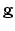. Such the uncertainty exists for any value of: since the time in GRT depends on the gravitational potential, then an effective potential will be nonzero even with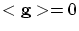. Whether is it possible to invent, though theoretically, a precise watch, which can be worn by anybody? Probably, a rotating flywheel with a mark (in the absence of friction - on a superconducting suspension), whose axis is directed along the gravitational field gradient (or along the resultant force for non-inertial systems) could read out the correct time. At least, no obvious reasons and mechanisms of changing the rotation rate are seen in this case. Certainly, for weak gravitation fields such a watch will be less accurate at the modern stage, than cesium one. Outside the criticism of relativity theory, we hypothesize, that atom decay is anisotropic, and this anisotropy can be interrelated with a direction of the atomic magnetic moment. In this case we can regulate atomic moments and freeze the system. Then, the "frozen clock" will register different time depending on its orientation in the gravitational field.

Now we return to synchronizing signals (for simultaneous measurement of lengths, for example). For a rectilinearly moving, accelerated system it is possible to use the signals from a remote source being perpendicular to the line of motion, and for the segment of a circle the source can be at its center. These cases actually cover all non-inertial motions without gravitation. (Besides, for the arbitrary planar motion it is possible to make use of a remote periodic source being on a perpendicular to the plane of motion.) For the real gravitational field of spherical bodies in arbitrary motion along the equipotential surfaces it is possible to use periodic signals issuing from the gravitational field center.

Note, that to prove the inconsistency of SRT and GRT conclusions on the change of lengths and time intervals it is sufficient, that the accuracy of ideal (classical) measurement of these values could principally exceed the value of the effect predicted by SRT and GRT. For example, for a synchronizing source being at the middle perpendicular to the line of motion we have for the precision of the time of synchronization: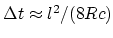, where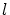is the length of a segments with the synchronized time,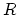is the distance to the synchronizing source; that is,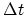can be decreased not only by choosing the great radius of a light sphere, but also by choosing a small section of motion. From the SRT formulas on time contraction we have for the similar value: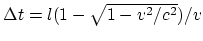. If for finiteand specified speed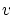we choose such, that the inequality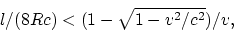(2.1)

be met, then the conclusions of relativistic theories occur to be invalid.

For the system arbitrarily moving along the radius (drawn from the gravitational field center) it is possible to use for synchronization a free falling periodic source on the perpendicular to the line of motion. In this caseshould be chosen of such value, that the field cannot actually change (due to equipotential sphere rounding) at this distance, and correspondingfrom (2.1) near the point, to which the perpendicular is drawn. Therefore, the GRT conclusions can be refuted in this case as well. For the most important special cases the "universal" SRT and GRT conclusions on the contraction of distances as a property of the space itself are invalid. In the most general case it seems intuitively quite obvious, that such a position of a periodic source can be found, that the signal to come perpendicular to the motion, and that suchandfrom (2.1) to exist, which refute the GRT results. There is no necessity at all in a "spread" frame of reference and in an arbitrarily operating clock: any change of real lengths should be explained by real forces; it is always possible to introduce a system of mutually motionless bodies and the universal time (even if it were the recalculation method). Thus, the space and time must be Newtonian and independent on the motion of a system.Next: Some GRT corollaries Up: Criticism of the GRT basis Previous: The equivalence principle   Contents
Sergey N. Arteha